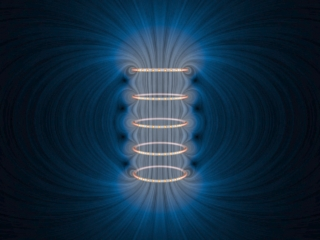| Visualizing EM | Vector Fields | Electrostatics | Magnetostatics | Faraday's Law | Light | Notes | Copyright |# SECTION : Creating Magnetic FieldsSUBJECT: Destroying a Magnetic Field DESCRIPTION: Suppose we have five rings that carry a number of free positive charges that are moving counter-clockwise. This current results in a magnetic field that is strong inside the rings and weak outside. Now suppose a set of external agents come along (one for each charge) and simultaneously spin down the charges as seen from above, at the same time and at the same rate, in a manner that has been pre-arranged. Once the charges on the rings start to deccelerate, the magnetic field begins to decrease in intensity. As the magnetic flux through the rings decreases, Faraday's Law tells us that there is an electric field induced by the time-changing magnetic field that is circulating clockwise as seen from above. The force on the charges due to this electric field is thus opposite the direction the external agents are trying to spin the rings down in (counter-clockwise), and thus work is done on those agents. As the strength of the magnetic field decreases, the magnetic energy flows from the field back to the path along which the charges move, and is now provided to the agents trying to spin down the moving charges. The energy provided to those agents as they destroy the magnetic field is exactly the amount of energy that they put into creating the magnetic field in the first place, neglecting radiative losses. This is a totally reversible process if we neglect such losses. That is, the amount of energy the agents put into creating the magnetic field is exactly returned to the agents as the field is destroyed. 640x480 version

 – Visualizing E&M – Vector Fields – Electrostatics – Magnetostatics – Faraday's Law – Light – Notes – Copyright –